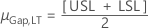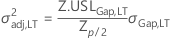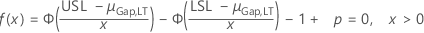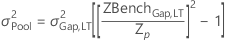# Calculations for the mean pool and the variance pool for Calculate Gap Pools

There are different calculations for the gap pools depending on whether you have specified shift or drift (variation expansion) factors for the elements and which gap specifications are given.

## Mean shift factor only

No gap specification

μPool = σGap,LT[Zp – Z.BenchGap,LT]

σ2Pool = 0

Lower gap specification only

μPool = σGap,LT[Zp – Z.BenchGap,LT]

σ2Pool = 0

Upper gap specification only

μPool = σGap,LT[Z.BenchGap,LT – Zp]

σ2Pool = 0

Both gap specifications

μPool = 0

* where if, thenor else σ2adj,LT is the unique solution to:## Variation expansion factor only, or both mean shift factor and variation expansion factor

One or no gap specification

μPool = 0Both gap specifications

μPool = 0

σ2Pool = σ2adj,LT – σ2Gap,LT *

###### Note

σ2Pool = 0 if T=LSL or T=USL and Zp=0

* where if, thenor else σ2adj,LT is the unique solution to:## Notation

TermDescription
CiDiametrical correction of the ith element
DiDrift factor for the ith element
NiComplexity of the ith element
SiShift factor for the ith element
σiStandard deviation of the ith element